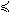## 17BMI Problem

The linear semi-definite programming problem with bilinear matrix inequalities (bmi) is defined similarly to SDP 18 but with the matrix inequality

Q0i +
 Σ k=1
n Qkixk +
 Σ k=1
n
 Σ l=1
n xkxlKkli0    (19)

The following file defines and solves a problem in TOMLAB.

File: tomlab/quickguide/bmiQG.m

Open the file for viewing, and execute bmiQG in Matlab.
```% bmiQG is a small example problem for defining and solving
% semi definite programming problems with bilinear matrix
% inequalities using the TOMLAB format.

Name='bmi.ps example 3';
A   = [];
b_U = [];
b_L = [];

c   = [ 0 0 1 ];  % cost vector

% One matrix constraint, set linear part first
SDP = [];

% The constant matrix is stored as
% SDP(i).Q{j} when SDP(i).Qidx(j) == 0
SDP(1).Q{1}  = [-10 -0.5 -2 ;-0.5 4.5 0 ;-2 0 0 ];

SDP(1).Q{2} = [ 9 0.5 0       ;  0.5  0  -3 ;  0  -3 -1 ];
SDP(1).Q{3} = [-1.8 -0.1 -0.4 ; -0.1 1.2 -1 ; -0.4 -1 0 ];

% Sparse is fine, too. Eventually, all the matrices are
% converted to sparse format.
SDP(1).Q{4} = -speye(3);

SDP(1).Qidx = [0; 1; 2; 3];

% Now bilinear part

% K_12 of constraint 1 (of 1) is nonzero, so set in SDP(i).K{1}.
SDP(1).K{1} = [0 0 2 ; 0 -5.5 3 ; 2 3 0 ];
SDP(1).Kidx = [1 2];
n   = length(c);

x_L = [-5 ; -3 ; -Inf];
x_U = [ 2 ;  7 ;  Inf];
x_0 = [ 0 ;  0 ;  0  ];

f_Low = [];

Prob = bmiAssign([], c, SDP, A, b_L, b_U, x_L, x_U, x_0,...
Name, f_Low);

Result = tomRun('penbmi', Prob, 1);```